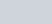In an A.P., the first term is 22, nth term is −11 and the sum
Question:

In an A.P., the first term is 22, nth term is −11 and the sum to first n terms is 66. Find n and d, the common difference

Solution:

In the given problem, we have the first and the nth term of an A.P. along with the sum of the n terms of A.P. Here, we need to find the number of terms and the common difference of the A.P.

Here,

The first term of the A.P (a) = 22

The nth term of the A.P (l) = −11

Sum of all the termsLet the common difference of the A.P. be d.

So, let us first find the number of the terms (n) using the formula,

$66=\left(\frac{n}{2}\right)[22+(-11)]$

$66=\left(\frac{n}{2}\right)(22-11)$

$(66)(2)=(n)(11)$

Further, solving for n

$n=\frac{(66)(2)}{11}$

$n=(6)(2)$

$n=12$

Now, to find the common difference of the A.P. we use the following formula,

$l=a+(n-1) d$

We get,

$-11=22+(12-1) d$

$-11=22+(11) d$

$\frac{-11-22}{11}=d$

Further, solving for d,

$d=\frac{-33}{11}$

$d=-3$

Therefore, the number of terms is $n=12$ and the common difference of the A.P. $d=-3$.